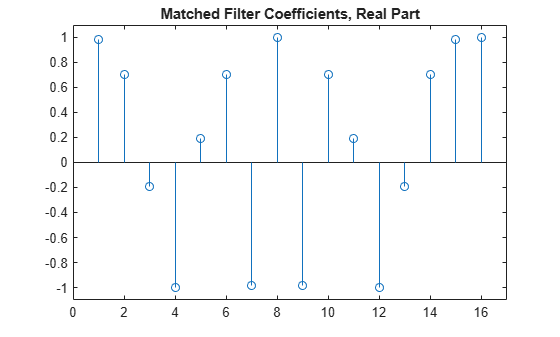# getMatchedFilter

System object: phased.PhaseCodedWaveform
Package: phased

Matched filter coefficients for waveform

## Syntax

```Coeff = getMatchedFilter(H) Coeff = getMatchedFilter(H,'FrequnecyOffset',FOFFSET) ```

## Description

`Coeff = getMatchedFilter(H)` returns the matched filter coefficients for the phase-coded waveform object, `H`. `Coeff` is a column vector.

`Coeff = getMatchedFilter(H,'FrequnecyOffset',FOFFSET)` adds a frequency offset when matched filter coefficients are generated. `FOFFSET` must be a positive scalar. This option is available when you set the `FrequencyOffsetSource` property to ```'Input port'``` for the input object, `H`.

## Input Arguments

 `H` Phase-coded waveform object.

## Output Arguments

 `Coeff` Column vector containing coefficients of the matched filter for `H`.

## Examples

expand all

Obtain the matched filter coefficients for a phase-coded pulse waveform that uses the Zadoff-Chu code.

```waveform = phased.PhaseCodedWaveform('Code','Zadoff-Chu','ChipWidth',1e-6, ... 'NumChips',16,'OutputFormat','Pulses','NumPulses',2); coeff = getMatchedFilter(waveform); stem(real(coeff)) title('Matched Filter Coefficients, Real Part') axis([0 17 -1.1 1.1])```